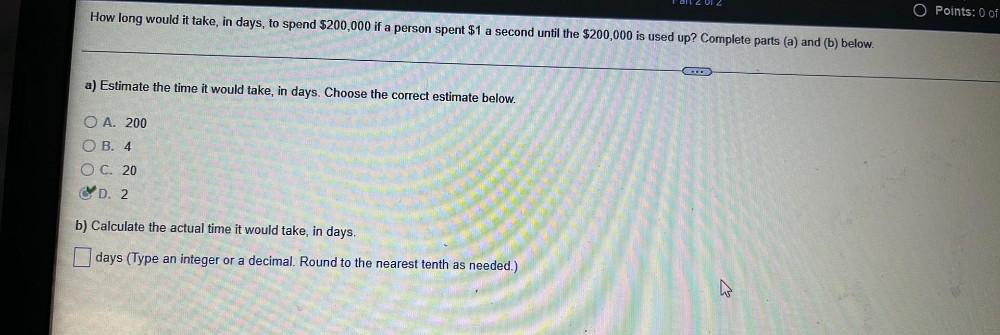Question:

# How long would it take, in days, to spend \$200,000 if a person spent \$1 a second until the \$200,000 is used up? Complete parts (a) and (b) below. a) Estimate the time it would take, in days. Choose thHow long would it take, in days, to spend \$200,000 if a person spent \$1 a second until the \$200,000 is used up? Complete parts (a) and (b) below. a) Estimate the time it would take, in days. Choose the correct estimate below. O A. 200 O B. 4 O C. 20 O D. 2 b) Calculate the actual time it would take, in days. □ days (Type an integer or a decimal. Round to the nearest tenth as needed.)